# forecastML Overview

#### 2020-05-05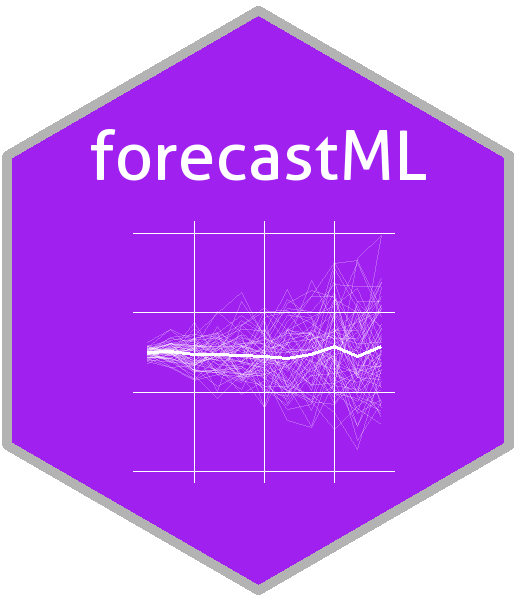# Purpose

The purpose of forecastML is to provide a series of functions and visualizations that simplify the process of multi-step-ahead forecasting with standard machine learning algorithms. It’s a wrapper package aimed at providing maximum flexibility in model-building–choose any machine learning algorithm from any R or Python package–while helping the user quickly assess the (a) accuracy, (b) stability, and (c) generalizability of grouped (i.e., multiple related time series) and ungrouped forecasts produced from potentially high-dimensional modeling datasets.

This package is inspired by Bergmeir, Hyndman, and Koo’s 2018 paper A note on the validity of cross-validation for evaluating autoregressive time series prediction. which supports–under certain conditions–forecasting with high-dimensional ML models without having to use methods that are time series specific.

The following quote from Bergmeir et al.’s article nicely sums up the aim of this package:

“When purely (non-linear, nonparametric) autoregressive methods are applied to forecasting problems, as is often the case (e.g., when using Machine Learning methods), the aforementioned problems of CV are largely irrelevant, and CV can and should be used without modification, as in the independent case.”

More information, cheat sheets, and worked examples can be found at https://github.com/nredell/forecastML.

# Forecasting Strategies in forecastML

For reference, below are some resources for learning more about multi-step-ahead forecasting strategies:

## Direct Forecasting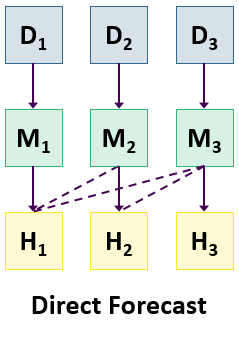In contrast to the recursive or iterated method for producing multi-step-ahead forecasts used in traditional forecasting methods like ARIMA, direct forecasting involves creating a series of distinct horizon-specific models. Though several hybrid methods exist for producing multi-step forecasts, the simple direct forecasting method used in forecastML lets us avoid the exponentially more difficult problem of having to “predict the predictors” for forecast horizons beyond 1-step-ahead.

The direct forecasting approach used in forecastML involves the following steps:

1. Build a series of horizon-specific short-, medium-, and long-term forecast models.

2. Assess model generalization performance across a variety of heldout datasets through time.

3. Select those models that consistently performed the best at each forecast horizon and combine them to produce a single ensemble forecast.

Below is a plot of 5 forecast models used to produce a single 12-step-ahead forecast where each color represents a distinct horizon-specific ML model. From left to right these models are:

1: A feed-forward neural network (purple); 2: An ensemble of ML models; 3: A boosted tree model; 4: A LASSO regression model; 5: A LASSO regression model (yellow).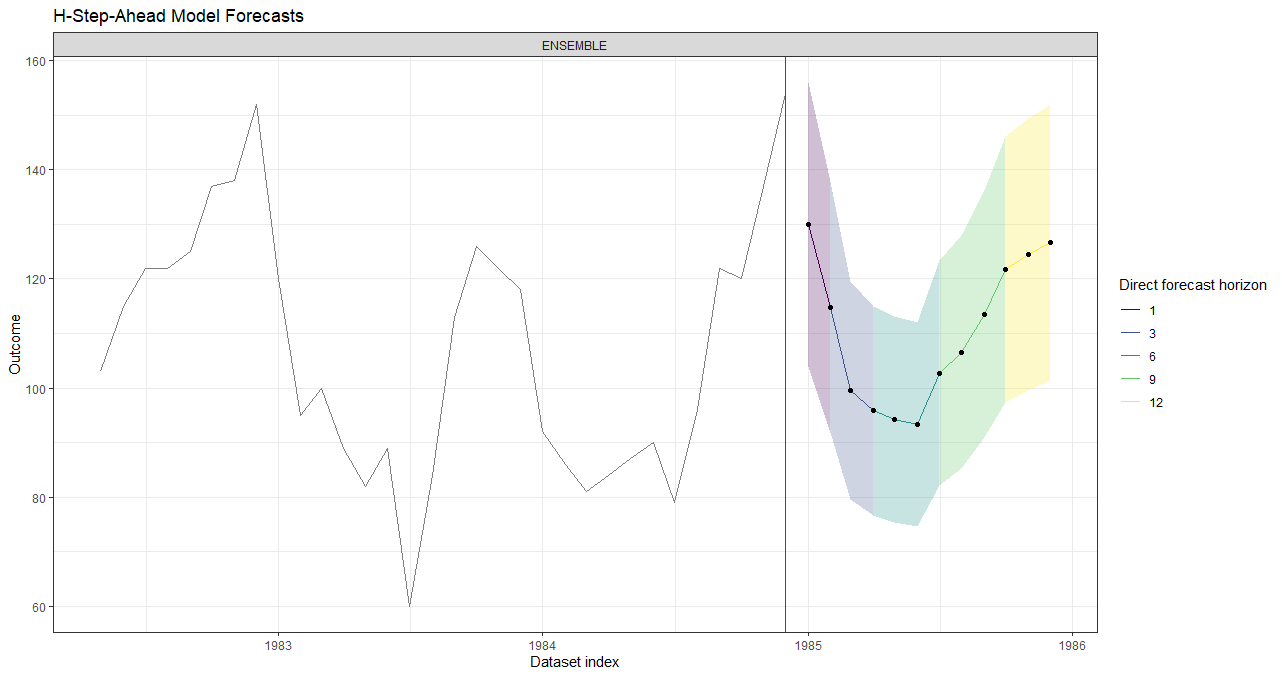## Multi-Output Forecasting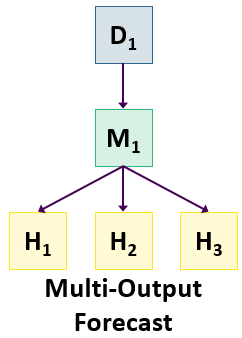The multi-output forecasting approach used in forecastML involves the following steps:

1. Build a single multi-output model that simultaneously forecasts over both short- and long-term forecast horizons.

2. Assess model generalization performance across a variety of heldout datasets through time.

3. Select the hyperparameters that minimize forecast error over the relevant forecast horizons and re-train.

# Key Functions

1. fill_gaps: Optional if no temporal gaps/missing rows in data collection. Fill gaps in data collection and prepare a dataset of evenly-spaced time series for modeling with lagged features. Returns a ‘data.frame’ with missing rows added in so that you can either (a) impute, remove, or ignore NAs prior to the forecastML pipeline or (b) impute, remove, or ignore them in the user-defined modeling function–depending on the NA handling capabilities of the user-specified model.

2. create_lagged_df: Create model training and forecasting datasets with lagged, grouped, dynamic, and static features.

3. create_windows: Create time-contiguous validation datasets for model evaluation.

4. train_model: Train the user-defined model across forecast horizons and validation datasets.

5. return_error: Compute forecast error across forecast horizons and validation datasets.

6. return_hyper: Return user-defined model hyperparameters across validation datasets.

7. combine_forecasts: Combine multiple horizon-specific forecast models to produce one forecast.

# Example - Direct Forecasting

In this walkthrough of forecastML we’ll compare the forecast performance of two machine learning methods, LASSO and Random Forest, across forecast horizons using the Seatbelts dataset from the datasets package.

Here’s a summary of the problem at hand:

• Outcome:
• DriversKilled - car drivers killed per month in the UK.
• Features:
• DriversKilled - car drivers killed per month in the UK.
• kms - a measure of distance driven.
• PetrolPrice - the price of gas.
• law - A binary indicator of the presence of a seatbelt law.
• Forecast:
• Build a 12-month-ahead forecast model as a combination of short- and long-term horizon-specific ML models.
• Model training - The first 15 years of the monthly dataset.
• Model testing - The last year of the monthly dataset.

## Install forecastML

install.packages("forecastML")

## Load Packages and Data

library(forecastML)
library(dplyr)
library(DT)
library(ggplot2)
library(glmnet)
library(randomForest)

data("data_seatbelts", package = "forecastML")
data <- data_seatbelts

date_frequency <- "1 month"  # Time step frequency.

# The date indices, which don't come with the stock dataset, should not be included in the modeling data.frame.
dates <- seq(as.Date("1969-01-01"), as.Date("1984-12-01"), by = date_frequency)

data$PetrolPrice <- round(data$PetrolPrice, 3)

data <- data[, c("DriversKilled", "kms", "PetrolPrice", "law")]
DT::datatable(head(data, 5))

## Train-Test Split

We’ll build our models on data_train and evaluate their out-of-sample performance on data_test.

data_train <- data[1:(nrow(data) - 12), ]
data_test <- data[(nrow(data) - 12 + 1):nrow(data), ]
p <- ggplot(data, aes(x = dates, y = DriversKilled))
p <- p + geom_line()
p <- p + geom_vline(xintercept = dates[nrow(data_train)], color = "red", size = 1.1)
p <- p + theme_bw() + xlab("Dataset index")
p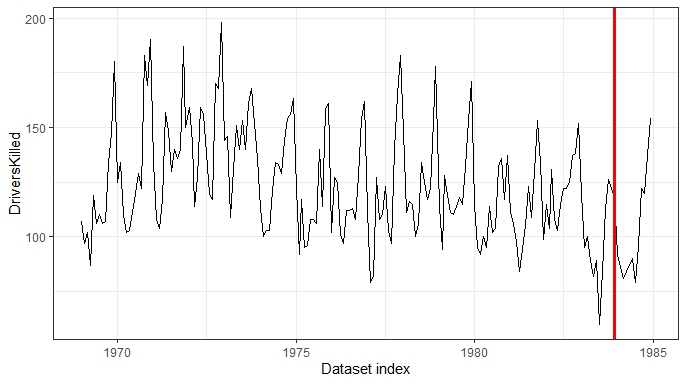## Data Preparation

### forecastML::create_lagged_df

We’ll create a list of datasets for model training, one for each forecast horizon.

outcome_col <- 1  # The column index of our DriversKilled outcome.

horizons <- c(1, 3, 6, 12)  # 4 models that forecast 1, 1:3, 1:6, and 1:12 time steps ahead.

# A lookback across select time steps in the past. Feature lags 1 through 9, for instance, will be
# silently dropped from the 12-step-ahead model.
lookback <- c(1:6, 9, 12, 15)

# A non-lagged feature that changes through time whose value we either know (e.g., month) or whose
# value we would like to forecast.
dynamic_features <- "law"

data_list <- forecastML::create_lagged_df(data_train,
outcome_col = outcome_col,
type = "train",
horizons = horizons,
lookback = lookback,
date = dates[1:nrow(data_train)],
frequency = date_frequency,
dynamic_features = dynamic_features
)

Let’s view the modeling dataset for a forecast horizon of 6. Notice that “lag” has been appended to all lagged features. Dynamic features keep their original names.

DT::datatable(head(data_list$horizon_6, 10), options = list(scrollX = TRUE)) The plot below illustrates, for a given lagged feature, the number and position (in dataset rows) of lagged features created for each forecast horizon/model. The lookback argument in created_lagged_df() was set to create lagged features from a minimum of 1 lag to a maximum of 15 lags; however, feature lags that don’t support direct forecasting at a given forecast horizon are silently removed from the modeling dataset. plot(data_list)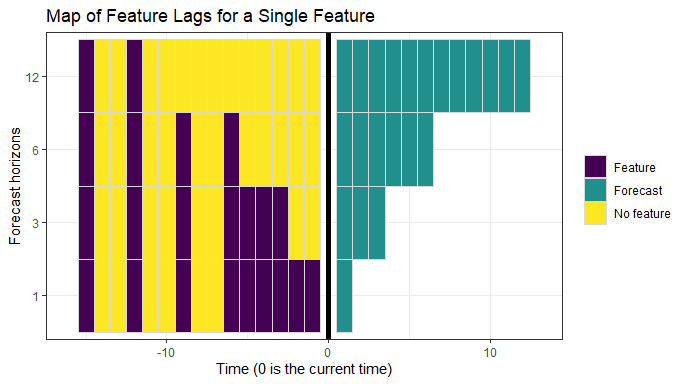## forecastML::create_windows create_windows() creates indices for partitioning the training dataset in the outer loop of a nested cross-validation setup. The validation datasets are created in contiguous blocks of window_length, as opposed to randomly selected rows, to mimic forecasting over multi-step-ahead forecast horizons. The skip, window_start, and window_stop arguments take dataset indices–or dates if a vector of dates is supplied to create_lagged_df()–that allow the user to adjust the number and placement of outer loop validation datasets. windows <- forecastML::create_windows(lagged_df = data_list, window_length = 12, skip = 48, window_start = NULL, window_stop = NULL, include_partial_window = TRUE) windows ## start stop window_length ## 1 1970-04-01 1971-03-01 12 ## 2 1975-04-01 1976-03-01 12 ## 3 1980-04-01 1981-03-01 12 Below is a plot of the nested cross-validation outer loop datasets or windows. In our example, a window_length of 12 (months) resulted in 3 validation windows. In this nested cross-validation setup, a model is trained with data from 2 windows and forecast accuracy is assessed on the left-out window. This means that we’ll need to train 3 models for each direct forecast horizon, each potentially selecting different optimal hyperparameters and having different coefficients–if available–from the inner cross-validation loop. Assessing the differences between these models is a good way to determine the stability of a given modeling approach under various time series dynamics. After model training and exploration, it’s entirely possible that a single multi-step-ahead forecast may use different ML algorithms (e.g., a neural network for shorter horizons and linear regression for longer horizons) to produce the short- and long-term forecasts. plot(windows, data_list, show_labels = TRUE)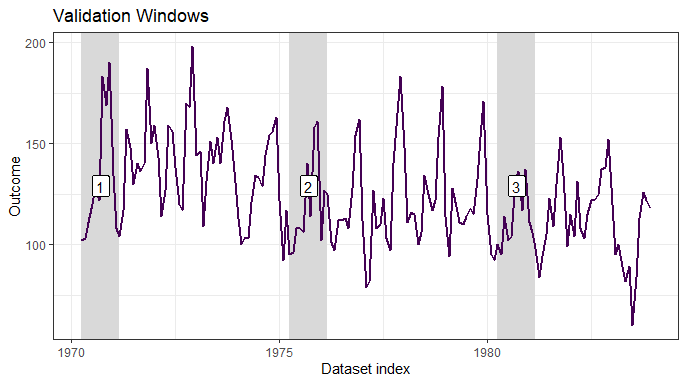## Model Training ### User-defined modeling function We’ll compare the forecasting performance of two models: (a) a cross-validated LASSO and (b) a non-tuned Random Forest. The following user-defined functions are needed for each model: • A user-defined wrapper function for model training that takes the following arguments: • 1: A horizon-specific data.frame made with create_lagged_df(..., type = "train") (e.g., my_lagged_df$horizon_h),
• 2: optionally, any number of additional named arguments which can be passed as ‘…’ in train_model() or set with default arguments in the model function.
• and returns a model object that will be passed into the user-defined predict() function.

Any data transformations, hyperparameter tuning, or inner loop cross-validation procedures should take place within this function, with the limitation that it ultimately needs to return() a model suitable for the user-defined predict() function; a list can be returned to capture meta-data and data pre-processing pipelines.

# Example 1 - LASSO
# Alternatively, we could define an outcome column identifier argument, say, 'outcome_col = 1' in
# this function or just 'outcome_col' and then set the argument as 'outcome_col = 1' in train_model().
model_function <- function(data) {

# The 'law' feature is constant during some of our outer-loop validation datasets so we'll
# simply drop it so that glmnet converges.
constant_features <- which(unlist(lapply(data[, -1], function(x) {!(length(unique(x)) > 1)})))

if (length(constant_features) > 1) {
data <- data[, -c(constant_features + 1)]  # +1 because we're skipping over the outcome column.
}

x <- data[, -(1), drop = FALSE]
y <- data[, 1, drop = FALSE]
x <- as.matrix(x, ncol = ncol(x))
y <- as.matrix(y, ncol = ncol(y))

model <- glmnet::cv.glmnet(x, y, nfolds = 3)
return(list("model" = model, "constant_features" = constant_features))
}

# Example 2 - Random Forest
# Alternatively, we could define an outcome column identifier argument, say, 'outcome_col = 1' in
# this function or just 'outcome_col' and then set the argument as 'outcome_col = 1' in train_model().
model_function_2 <- function(data) {

outcome_names <- names(data)
model_formula <- formula(paste0(outcome_names,  "~ ."))

model <- randomForest::randomForest(formula = model_formula, data = data, ntree = 200)
return(model)
}

### forecastML::train_model

For each modeling approach, LASSO and Random Forest, a total of N forecast horizons * N validation windows models are trained. In this example, that means training 12 models for each algorithm.

These models could be trained in parallel on any OS with the very flexible future package by un-commenting the code below and setting use_future = TRUE. To avoid nested parallelization, models are either trained in parallel across forecast horizons or validation windows, whichever is longer (when equal, the default is parallel across forecast horizons).

#future::plan(future::multiprocess)

model_results <- forecastML::train_model(data_list, windows, model_name = "LASSO",
model_function, use_future = FALSE)

model_results_2 <- forecastML::train_model(data_list, windows, model_name = "RF",
model_function_2, use_future = FALSE)

### User-defined prediction function

The following user-defined prediction function is needed for each model:

• A wrapper function that takes the following 2 positional arguments:
• 1: The model returned from the user-defined modeling function.
• 2: A data.frame of the model features from create_lagged_df(..., type = "train").
• and returns a data.frame of predictions with 1 or 3 columns. A 1-column data.frame will produce point forecasts, and a 3-column data.frame can be used to return point, lower, and upper forecasts (column names and order do not matter).
# Example 1 - LASSO.
prediction_function <- function(model, data_features) {

if (length(model$constant_features) > 1) { # 'model' was passed as a list. data_features <- data_features[, -c(model$constant_features )]
}

x <- as.matrix(data_features, ncol = ncol(data_features))

data_pred <- data.frame("y_pred" = predict(model$model, x, s = "lambda.min")) return(data_pred) } # Example 2 - Random Forest. prediction_function_2 <- function(model, data_features) { data_pred <- data.frame("y_pred" = predict(model, data_features)) return(data_pred) } ### Predict on historical data The predict.forecast_model() S3 method takes any number of trained models from train_model() and a list of user-defined prediction functions. The list of prediction functions should appear in the same order as the models. Note that the prediction_function and data arguments need to be named because the first function argument is .... Outer loop nested cross-validation forecasts are returned for each user-defined model, forecast horizon, and validation window. data_results <- predict(model_results, model_results_2, prediction_function = list(prediction_function, prediction_function_2), data = data_list) Let’s view the models’ predictions. data_results$DriversKilled_pred <- round(data_results$DriversKilled_pred, 0) DT::datatable(head(data_results, 30), options = list(scrollX = TRUE)) Below is a plot of the historical forecasts for each validation window at select forecast horizons. plot(data_results, type = "prediction", horizons = c(1, 6, 12))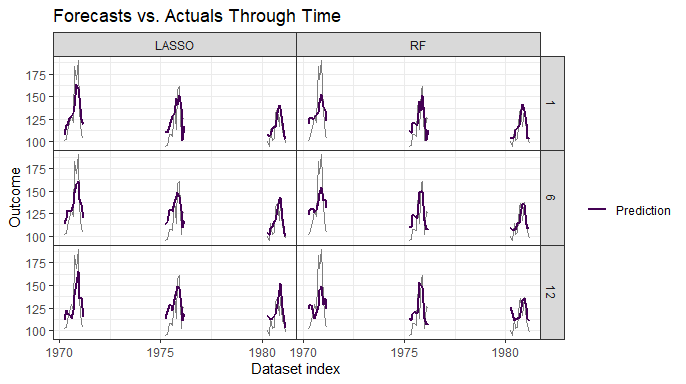Below is a plot of the historical forecast error for select validation windows at select forecast horizons. plot(data_results, type = "residual", horizons = c(1, 6, 12))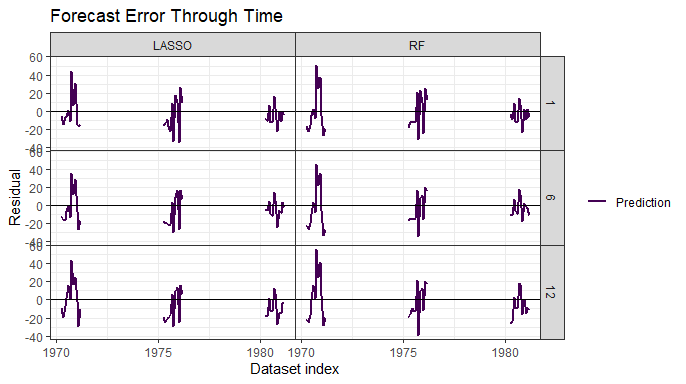The plot below is a diagnostic plot to check how forecasts for a target point in time have changed through time by looking at a history of forecasts. In this example, we have 4 direct forecast horizons–1, 3, 6, 12–so each of the colored points represents the origin of the forecast for the black point. In most cases it would be reasonable to expect shorter-horizon forecasts to be more accurate than longer-horizon forecasts. • Plot: Rolling origin forecasts for the last validation window in our training data. plot(data_results, type = "forecast_stability", windows = 3)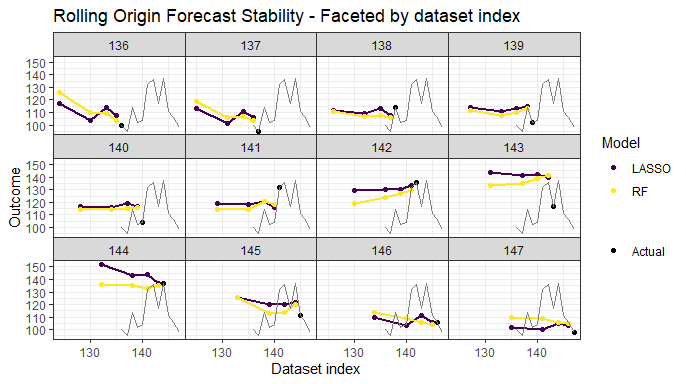## Model Performance ### forecastML::return_error Let’s calculate several common forecast error metrics for our holdout data sets in the training data. The forecast errors for nested cross-validation are returned at 3 levels of granularity: 1. Error by validation window 2. Error by model forecast horizon, collapsed across validation windows (only useful when multiple direct forecast horizons are trained together) 3. Global error collapsed across validation windows and model forecast horizons data_error <- forecastML::return_error(data_results) # Global error. data_error$error_global[, -1] <- lapply(data_error$error_global[, -1], round, 1) DT::datatable(data_error$error_global, options = list(scrollX = TRUE), )

Below is a plot of error metrics across time for select validation windows and forecast horizons.

plot(data_error, type = "window", facet = ~ horizon, horizons = c(1, 6, 12))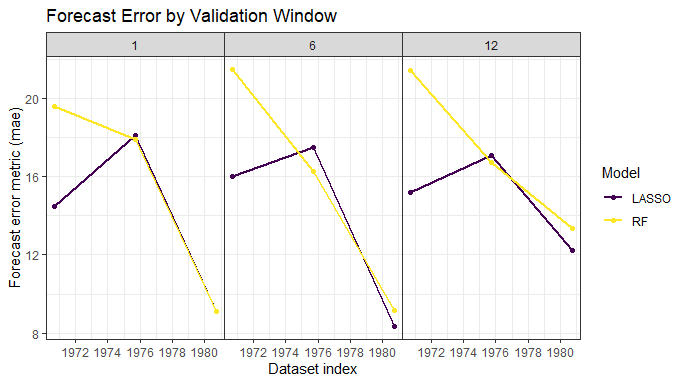Below is a plot of forecast error metrics by forecast model horizon collapsed across validation windows.

plot(data_error, type = "horizon", facet = ~ horizon, horizons = c(1, 6, 12))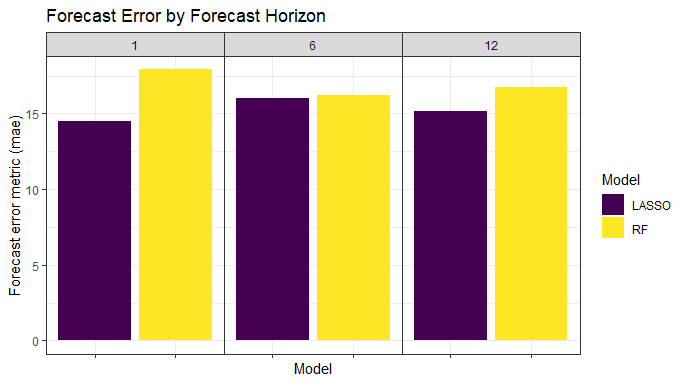Below is a plot of error metrics collapsed across validation windows and forecast horizons.

plot(data_error, type = "global", facet = ~ horizon)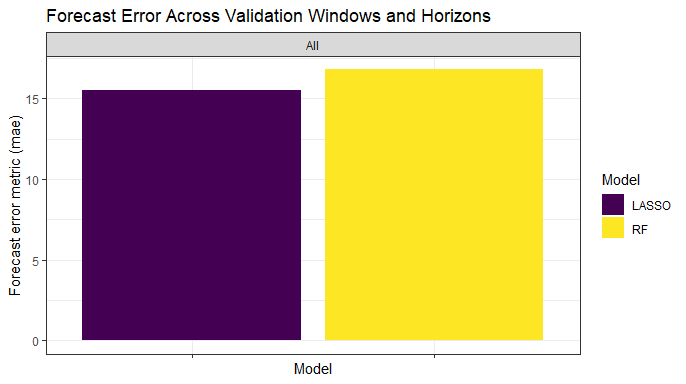## Hyperparameters

While it may be reasonable to have distinct models for each forecast horizon or even forecasting model ensembles across horizons, at this point we still have slightly different LASSO and Random Forest models from the outer loop of the nested cross-validation within each horizon-specific model. Here, we’ll take a look at the stability of the hyperparameters for the LASSO model to better understand if we can train one model across forecast horizons or if we need additional predictors or modeling strategies to forecast well under various conditions or time series dynamics.

### User-defined hyperparameter function

The following user-defined hyperparameter function is needed for each model:

• A wrapper function that takes the following 1 positional argument
• 1: The model returned from the user-defined modeling function.
• and returns a 1-row data.frame.
hyper_function <- function(model) {

lambda_min <- model$model$lambda.min
lambda_1se <- model$model$lambda.1se

data_hyper <- data.frame("lambda_min" = lambda_min, "lambda_1se" = lambda_1se)
return(data_hyper)
}

### forecastML::return_hyper

Below are two plots which show (a) univariate hyperparameter variability across the training data and (b) the relationship between each error metric and hyperparameter values.

data_hyper <- forecastML::return_hyper(model_results, hyper_function)

plot(data_hyper, data_results, data_error, type = "stability", horizons = c(1, 6, 12))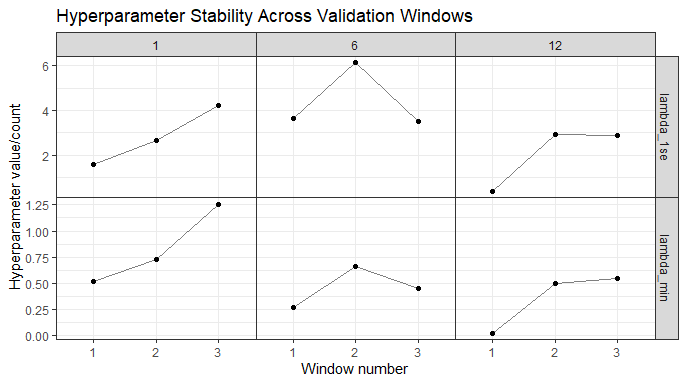plot(data_hyper, data_results, data_error, type = "error", c(1, 6, 12))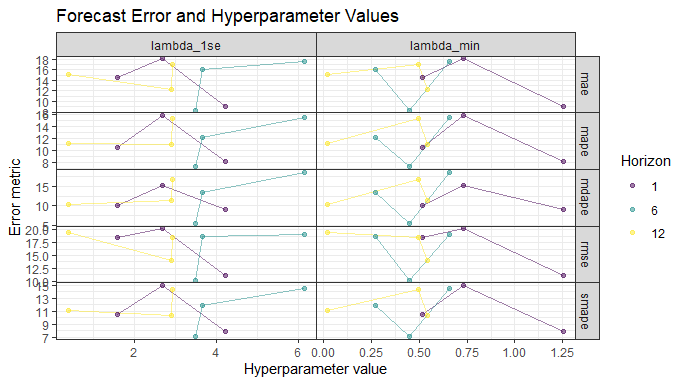## Forecast with Multiple Models from Nested CV

### forecastML::create_lagged_df

To forecast with the direct forecasting method, we need to create another dataset of forward-looking features. We can do this by running create_lagged_df() and setting type = "forecast".

Below is the forecast dataset for a 6-step-ahead forecast.

The forecast dataset has the following columns:

• index: A column giving the row index or date of the forecast periods (e.g., a 100 row non-date-based training dataset would start with an index of 101).
• horizon: A column that indicates the forecast period from 1:max(horizons).
• “features”: Lagged, dynamic, group, and static features identical to the type = "train", dataset.
data_forecast_list <- forecastML::create_lagged_df(data_train,
outcome_col = outcome_col,
type = "forecast",
horizons = horizons,
lookback = lookback,
date = dates[1:nrow(data_train)],
frequency = date_frequency,
dynamic_features = dynamic_features
)

DT::datatable(head(data_forecast_list$horizon_6), options = list(scrollX = TRUE)) ### Dynamic features Because we didn’t treat law as a lagged feature, we’ll have to fill in its future values when direct forecasting 1, 3, 6, and 12 steps ahead. In this example, we know that law <- 1 for the next 12 months. If we did not know the future values of law we would either have to use a class of models that can predict with missing features or forecast the value of law 1:12 months ahead. for (i in seq_along(data_forecast_list)) { data_forecast_list[[i]]$law <- 1
}

### Forecast results

Running the predict method, predict.forecast_model(), on the dataset created above–with type = "forecast"–and placing it in the data argument in predict.forecast_model() below, returns a data.frame of forecasts.

An S3 object of class, forecast_results, is returned. This object will have different plotting and error methods than the training_results class from earlier.

data_forecast <- predict(model_results, model_results_2,  # ... supports any number of ML models.
prediction_function = list(prediction_function, prediction_function_2),
data = data_forecast_list)

data_forecast$DriversKilled_pred <- round(data_forecast$DriversKilled_pred, 0)

DT::datatable(head(data_forecast, 10), options = list(scrollX = TRUE))

Below is a plot of the forecasts vs. the actuals for each model at select forecast horizons.

Setting the data_actual = ... and actual_indices = ... arguments plots a background dataset (gray line in the plots below).

It’s clear from the plots that our Random Forest model is producing less accurate forecasts and is more sensitive to the data on which it was trained.

plot(data_forecast,
data_actual = data[-(1:150), ],  # Actuals from the training and test data sets.
actual_indices = dates[-(1:150)],
horizons = c(1, 6, 12))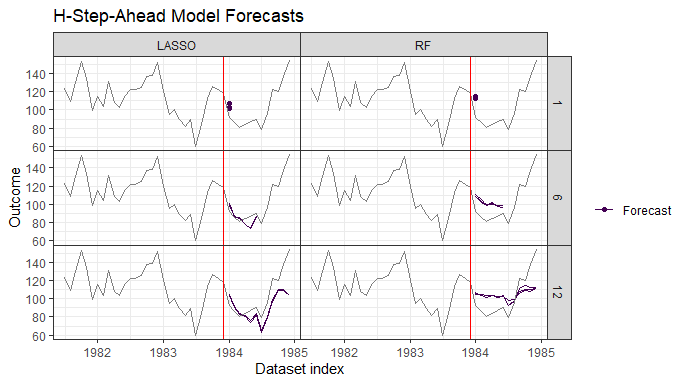## Forecast Error

### forecastML::return_error

Finally, we’ll look at our out-of-sample forecast error by forecast horizon for our two models by setting data_test = data_test.

If the first argument of return_error() is an object of class forecast_results and the data_test argument is a data.frame like data_test from our beginning train-test split, a data.frame of forecast error metrics with the following columns is returned:

• model: User-supplied model name in train_model().
• model_forecast_horizon: The direct-forecasting time horizon that the model was trained on.
• “error_metrics”: Forecast error metrics.

Below are 3 forecast error plots at various levels of aggregation.

data_error <- forecastML::return_error(data_forecast,
data_test = data_test,
test_indices = dates[(nrow(data_train) + 1):length(dates)])

plot(data_error, facet = ~ horizon, type = "window")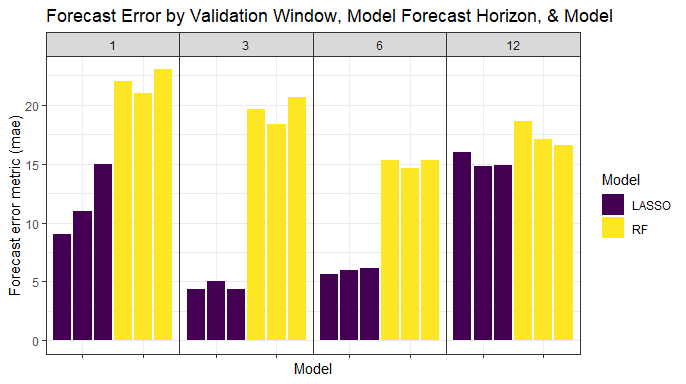plot(data_error, facet = ~ horizon, type = "horizon")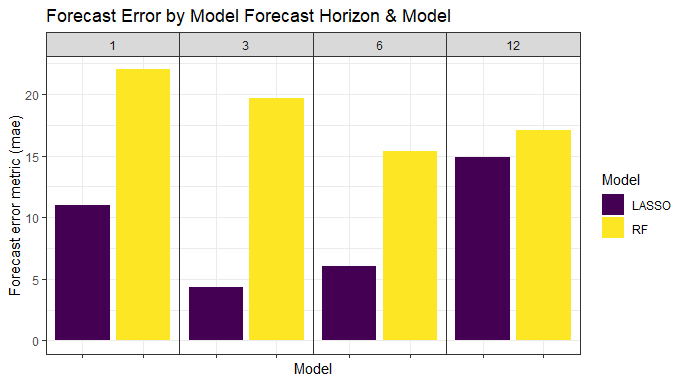plot(data_error, facet = ~ horizon, type = "global")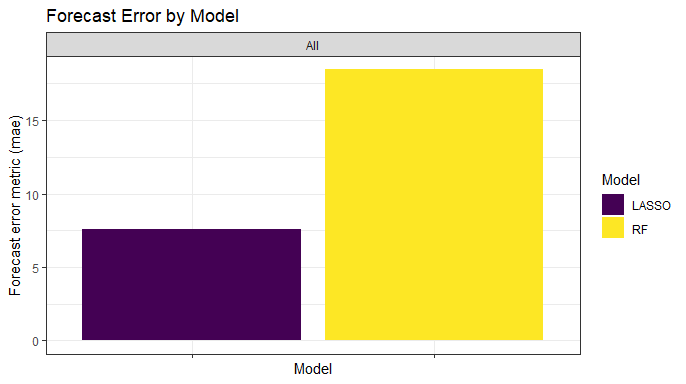## Model Selection and Re-training

Because our LASSO model is both more stable and accurate, we’ll re-train this model across the entire training dataset to get our final 4 models–1 for each forecast horizon. Note that for a real-world forecasting problem this is when we would do additional model tuning to improve forecast accuracy across validation windows as well as narrow the hyperparameter search in the user-specified modeling functions.

data_list <- forecastML::create_lagged_df(data_train,
outcome_col = outcome_col,
type = "train",
horizons = horizons,
lookback = lookback,
date = dates[1:nrow(data_train)],
frequency = date_frequency,
dynamic_features = dynamic_features
)

To create a dataset without nested cross-validation, set window_length = 0 in create_windows().

windows <- forecastML::create_windows(data_list, window_length = 0)

plot(windows, data_list, show_labels = TRUE)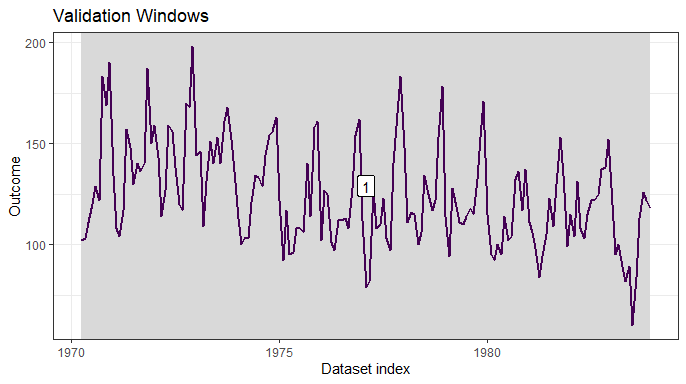Without nested cross-validation and holdout windows, the prediction plot is essentially a plot of model fit.

model_results <- forecastML::train_model(data_list, windows,  model_name = "LASSO", model_function)

data_results <- predict(model_results, prediction_function = list(prediction_function), data = data_list)

DT::datatable(head(data_results, 10), options = list(scrollX = TRUE))
plot(data_results, type = "prediction", horizons = c(1, 6, 12))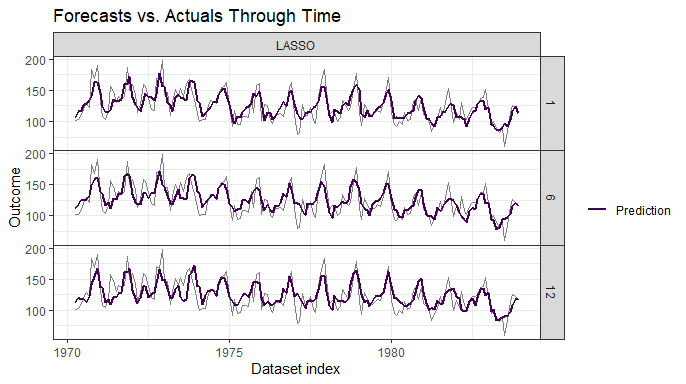### Training error with forecastML::return_error

Below is a the training error collapsed across our 4 direct forecast horizons/models.

data_error <- forecastML::return_error(data_results)

data_error$error_global[, -1] <- lapply(data_error$error_global[, -1], round, 1)

DT::datatable(head(data_error$error_global), options = list(scrollX = TRUE)) ## Forecast with 1 Model Per Horizon data_forecast_list <- forecastML::create_lagged_df(data_train, outcome_col = outcome_col, type = "forecast", horizons = horizons, lookback = lookback, date = dates[1:nrow(data_train)], frequency = date_frequency, dynamic_features = dynamic_features ) for (i in seq_along(data_forecast_list)) { data_forecast_list[[i]]$law <- 1
}

data_forecast <- predict(model_results, prediction_function = list(prediction_function), data = data_forecast_list)

plot(data_forecast,
data_actual = data[-(1:150), ],
actual_indices = dates[-(1:150)],
horizons = c(1, 6, 12))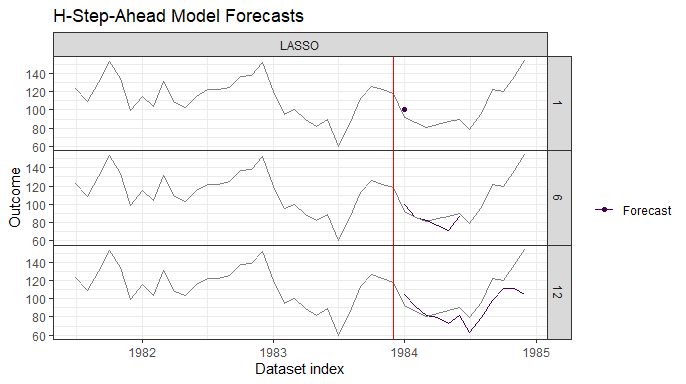### Forecast error with forecastML::return_error

data_error <- forecastML::return_error(data_forecast, data_test = data_test,
test_indices = dates[(nrow(data_train) + 1):nrow(data)])

plot(data_error, type = "horizon", facet = ~ horizon)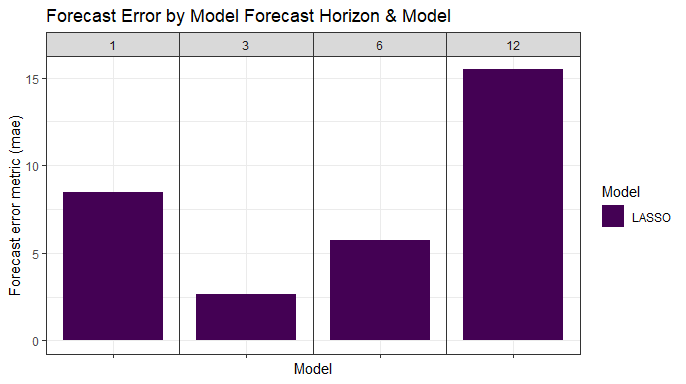## Forecast Combination with forecastML::combine_forecasts

• The final step in the forecastML framework is to combine multiple direct-horizon forecast models with combine_forecasts() to produce a single h-step-ahead forecast.

• The default approach, type = 'horizon', is to combine forecasts across models such that short-term models produce the shorter-term forecasts and long-term models produce the longer-term forecasts. This implies that, for our 12-month-ahead forecast,

• the 1-step-ahead model forecasts the next month,
• the 3-step-ahead model forecasts from months 2 through 3,
• the 6-step-ahead model forecasts from months 4 through 6, and
• the 12-step-ahead model forecasts from months 7 through 12.
data_combined <- forecastML::combine_forecasts(data_forecast)

# Plot a background dataset of actuals using the most recent data.
data_actual <- data[dates >= as.Date("1980-01-01"), ]
actual_indices <- dates[dates >= as.Date("1980-01-01")]

# Plot all final forecasts plus historical data.
plot(data_combined, data_actual = data_actual, actual_indices = actual_indices)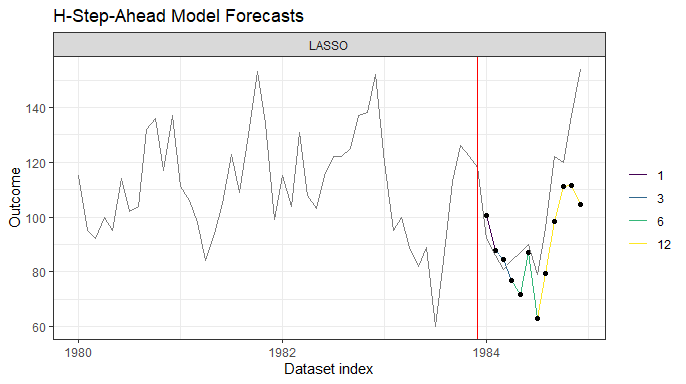# Error by forecast horizon.
DT::datatable(return_error(data_combined, data_actual, actual_indices)$error_by_horizon, options = list(scrollX = TRUE)) # Error aggregated across forecast horizons. DT::datatable(return_error(data_combined, data_actual, actual_indices)$error_global,
options = list(scrollX = TRUE))